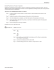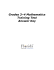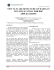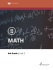# WISCONSIN MIDDLE SCHOOL STATE MATHEMATICS MEET WISCONSIN MATHEMATICS COUNCIL Sample Questions

## Transcription

WISCONSIN MIDDLE SCHOOL STATE MATHEMATICS MEET WISCONSIN MATHEMATICS COUNCIL Sample Questions
```WISCONSIN MIDDLE SCHOOL STATE MATHEMATICS MEET
WISCONSIN MATHEMATICS COUNCIL
Sample Questions
Problem Set #1
[Reduce all common fractions. Decimal approximations are not accepted except
when specifically asked for. When allowed, round decimal approximations to 3
decimal places. No rounding should be done except on the final answer.]
1. (1 point)
Three standard dice are rolled. What is the
probability of rolling a 1 on all three dice?
2. (3 points)
Two identical squares with sides
of length 10 cm overlap to form
a shaded region as shown. A
corner of one square lies at the
intersection of the diagonals of
the other square. Find the area
of the shaded region in cm2.
3. (5 points)
Dave is three times as old as Jim, and Jim is half
as old as Tom. If their average age is 64 years old,
how old is Jim?
cm2
WISCONSIN MIDDLE SCHOOL STATE MATHEMATICS MEET
WISCONSIN MATHEMATICS COUNCIL
Sample Questions
Problem Set #2
[Reduce all common fractions. Decimal approximations are not accepted except
when specifically asked for. When allowed, round decimal approximations to 3
decimal places. No rounding should be done except on the final answer.]
1. (1 point)
ABCD is a rectangle as shown. A
E lies on side AD , and ∠BEC is
a right angle of ∆BEC. CE = 6,
BE = 8, and BC = 10. Find the
B
area of rectangle ABCD.
E
8
D
6
10
C
un2
2. (3 points)
There are 30 students on the Euclid math team. 18 of
them took Algebra and 15 of them took Geometry,
while 4 of them took neither Algebra nor Geometry.
How many students took Algebra but not Geometry?
3. (5 points)
There is exactly one pair of numbers (x, y) for which
both x + 2y = 3 and 4x + 5y = 6. In this pair of
equations, what are x and y?
(x, y) = (______, ______)
WISCONSIN MIDDLE SCHOOL STATE MATHEMATICS MEET
WISCONSIN MATHEMATICS COUNCIL
Sample Questions
Team Problem Set
[Reduce all common fractions. Decimal approximations are not accepted except
when specifically asked for. When allowed, round decimal approximations to 3
decimal places. No rounding should be done except on the final answer.]
1. (10 points)
The Math Magic Middle School has 900 students.
Each student takes 7 classes per day, and each
teacher teaches 5 classes per day. Each class
contains 1 teacher and 20 students. How many
teachers does the Math Magic Middle School have?
2. (10 points)
In the diagram below, you can trace a “path” from left
to right that spells the word GEOMETRY correctly.
How many different paths exist in the diagram?
T
E
O
G
E
M
E
O
R
T
E
Y
R
T
```

### Page 1 Proving a triangle is a right triangle Method 1: Show two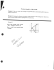### Math Wuzzles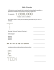### Wisconsin TEAM BEEF - Wisconsin Beef Council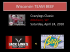### Dewey Decimal Classification System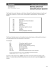### MATH 148 FINAL EXAM SAMPLE PROBLEMS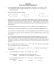### MAT.HS.CR.1.00FIF.L.614 HS Mathematics Sample CR Item C1 TL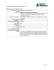### 11.4 So what is this thing called pi? student activity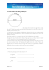### Sample MidTerm Exam Solutions - ENGG1811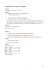### Section 1.1 Square Roots of Perfect Squares A) 0.6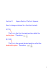### Stat 2610 Test 2 Review Names For full credit circle answers and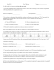### C3 Worksheet C - A Level Maths Help### Sig. Fig PPT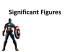### 1. S.I. unit 10. Extensive 19. Homogeneous Mixture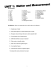### Proficiency Exam Sample Questions and Answers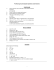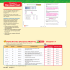### Ch 2 Measurements SI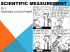### Lesson 6: Finite and Infinite Decimals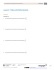### 1.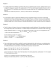### book here